SLOS190H February   1997  – March 2016

PRODUCTION DATA.

1. Features
2. Applications
3. Description
4. Revision History
5. Pin Configuration and Functions
6. Specifications
7. Detailed Description
8. Application and Implementation
1. 8.1 Application Information
2. 8.2 Typical Application
1. 8.2.1 High-Side Current Monitor
9. Power Supply Recommendations
10. 10Layout
11. 11Device and Documentation Support
12. 12Mechanical, Packaging, and Orderable Information

• D|8
• PW|8
• P|8
• D|8

## 8 Application and Implementation

NOTE

Information in the following applications sections is not part of the TI component specification, and TI does not warrant its accuracy or completeness. TI’s customers are responsible for determining suitability of components for their purposes. Customers should validate and test their design implementation to confirm system functionality.

### 8.1 Application Information

#### 8.1.1 Macromodel Information

Macromodel information provided was derived using MicroSim Parts™, the model generation software used with MicroSim PSpice™. The Boyle macromodel (1) and subcircuit in Figure 59 were generated using the TLC227x typical electrical and operating characteristics at TA = 25°C. Using this information, output simulations of the following key parameters can be generated to a tolerance of 20% (in most cases):

• Maximum positive output voltage swing
• Maximum negative output voltage swing
• Slew rate
• Quiescent power dissipation
• Input bias current
• Open-loop voltage amplification
• Unity gain frequency
• Common-mode rejection ratio
• Phase margin
• DC output resistance
• AC output resistance
• Short-circuit output current limit

(1)Macromodeling of Integrated Circuit Operational Amplifiers, IEEE Journal of Solid-State Circuits, SC-9, 353 (1974).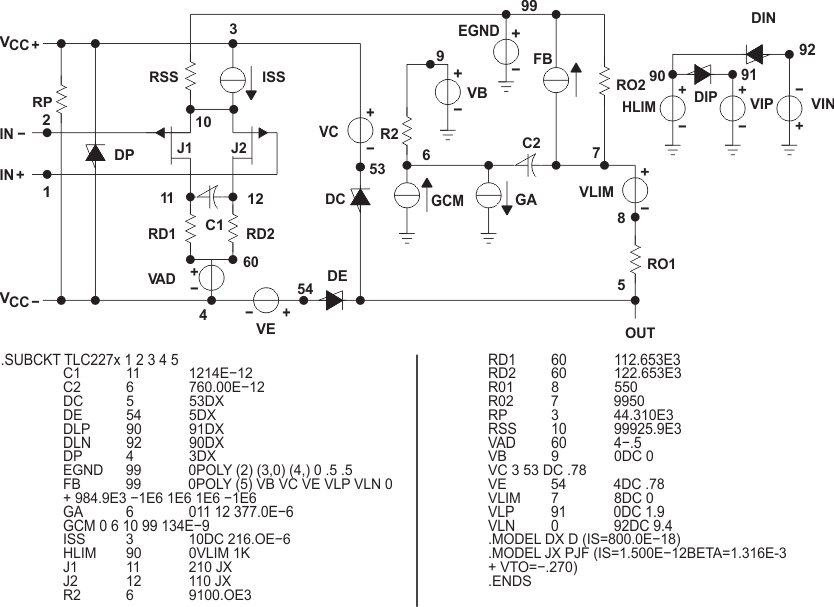Figure 59. Boyle Macromodel and Subcircuit

### 8.2 Typical Application

#### 8.2.1 High-Side Current MonitorFigure 60. Equivalent Schematic (Each Amplifier)

#### 8.2.1.1 Design Requirements

For this design example, use the parameters listed in Table 3 as the input parameters.

### Table 3. Design Parameters

PARAMETER VALUE
VBAT Battery Voltage 12 V
RSENSE Sense Resistor 0.1 Ω
Operational Amplifier Set in Differential configuration with Gain = 10

#### 8.2.1.2 Detailed Design Procedure

This circuit is designed for measuring the high-side current in automotive body control modules with 12-V battery or similar applications. The operational amplifier is set as differential with an external resistor network.

#### 8.2.1.2.1 Differential Amplifier Equations

Equation 1 and Equation 2 are used to calculate VOUT.

Equation 1.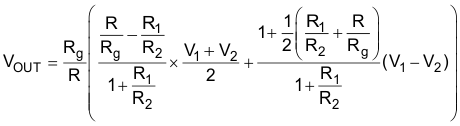Equation 2.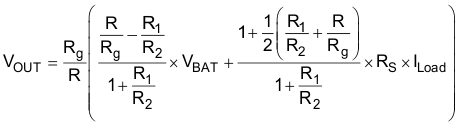In an ideal case R1 = R and R2 = Rg, and VOUT can then be calculated using Equation 3:

Equation 3.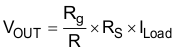However, as the resistors have tolerances, they cannot be perfectly matched.

R1 = R ± ΔR1

R2 = R2 ± ΔR2

R = R ± ΔR

Rg = Rg ± ΔRg

Equation 4.By developing the equations and neglecting the second order, the worst case is when the tolerances add up. This is shown by Equation 5.

Equation 5.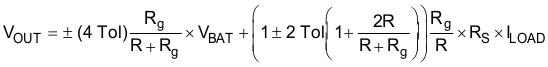where

• Tol = 0.01 for 1%
• Tol = 0.001 for 0.1%

If the resistors are perfectly matched, then Tol = 0 and VOUT is calculated using Equation 6.

Equation 6.The highest error is from the Common mode, as shown in Equation 7.

Equation 7.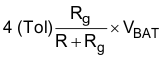Gain of 10, Rg / R = 10, and Tol = 1%:

Common mode error = ((4 × 0.01) / 1.1) × 12 V = 0.436 V

Gain of 10 and Tol = 0.1%:

Common mode error = 43.6 mV

The resistors were chosen from 2% batches.

R1 and R 12 kΩ

R2 and Rg 120 kΩ

Ideal Gain = 120 / 12 = 10

The measured value of the resistors:

R1 = 11.835 kΩ

R = 11.85 kΩ

R2 = 117.92 kΩ

Rg = 118.07 kΩ

#### 8.2.1.3 Application Curves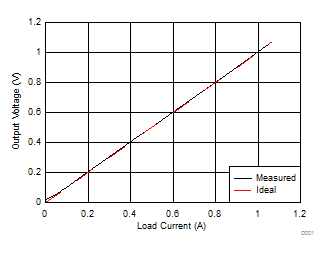Figure 61. Output Voltage Measured vs Ideal
(0 to 1 A)Figure 62. Output Voltage Measured vs Ideal
(0 to 10 A)